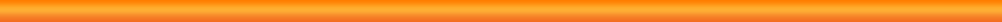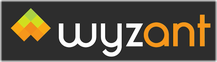# BooksSubject Description Schaum's Solved Problem Series Calculus Schaum's 3000 Solved Problems in Calculus (2009) Linear Algebra Schaum's 3000 Solved Problems in Linear Algebra Discrete Mathematics Schaum's 2000 Solved Problems in Discrete Mathematics Physics Schaum's 3000 Solved Problems in Physics (1988) Chemistry Schaum's 3000 Solved Problems in Chemistry Schaum's Outlines Precalculus Schaum's Outline of Precalculus, 2nd Edition (2008) Advanced Calculus Schaum's Outline of Advanced Calculus, 3rd Edition (2010) Differential Equations Schaum's Outline of Differential Equations, 3rd Edition Exams The Real ACT Prep Guide Peterson's, ACT Inc., 2nd Edition (2008) 10 ACT Practice Tests McGraw-Hill, 3rd Edition (2010) Cracking the ACT, 2011 Edition The Princton Review, 2011 Edition (2010) The Official SAT Study Guide for ALL Subject Tests CollegeBoard, 2nd Edition (2011) Kaplan SAT 2011: Strategies, Practice, and Review Kaplan (2010) 10 Real SATs The College Board, 3rd Edition (2003) THEA Test (formerly TASP) The Official THEA Test Study Guide Math Table Textbooks CRC Standard Mathematical Tables and Formulae Daniel Zwillinger, 31st Edition (2002) CRC Standard Mathematical Tables 27th Edition, William H. Beyer, Ph.D. (1984) Paul's Online Math Textbooks Algebra Text Book Free Algebra textbook Complex Number Primer Free Complex Numbers textbook Calculus I Text Book Free Calculus I textbook Calculus II Text Book Free Calculus II textbook Calculus III Text Book Free Calculus III textbook Linear Algebra Text Book Free Linear Algebra textbook Differential Equations Text Book Free Differential Equations textbook Calculus Textbooks Calculus (Larson) Calculus, Ron Larson and Bruce Edwards, 9th Edition (2009) - Link Calculus 9th Edition - Ron Larson & Bruce Edwards (PDF) Calculus, Ron Larson and Bruce Edwards, 9th Edition (2009) - Text Book Calculus - Solutions Guide - Online Calculus, Ron Larson and Bruce Edwards, 9th Edition (2009) - Solutions Guide - Online Calculus - Solutions Guide - CalcChat iPhone App Calculus, Ron Larson and Bruce Edwards, 9th Edition (2009) - Solutions Guide - CalcChat iPhone App Student Solutions Manual, Volume 1 for Larson/Edwards' Calculus, 9th Bruce Edwards (2008) Student Solutions Manual, Volume 2 for Larson/Edwards' Calculus, 9th Bruce Edwards (2009) Calculus (Stewart) Calculus, James Stewart, 7th Edition (2012) - Link Stewart's Single Variable Calculus Early Transcendentals, 7th (PDF) Calculus, James Stewart, 7th Edition (2012) - Text Book (PDF) Student Solutions Manual for Stewart's Single Variable Calculus Early Transcendentals, 7th (PDF) Calculus, James Stewart, 7th Edition (2012) - Solutions Guide (PDF) Schaum's Solved Problem Series - Calculus Schaum's 3000 Solved Problems in Calculus (2009) Schaum's Outlines - Advanced Calculus Schaum's Outline of Advanced Calculus, 3rd Edition (2010) Alternate Edition Calculus with analytic geometry Earl William Swokowski, 1st Edition (1983) Calculus Stanley I. Grossman, Calculus, 2nd Edition (1981) Precalculus Textbooks Precalculus: Graphical, Numerical, Algebraic Franklin Demana, Bert K. Waits, Gregory D. Foley and Daniel Kennedy, 7th Edition (2006) Numerical Analysis Textbooks Numerical Analysis, 4th Edition Authors: Richard L. Burden & J. Douglas Faires, Size: 729 pages, Date: 1988 Numerical Analysis, 9th Edition Authors: Richard L. Burden & J. Douglas Faires, Size: 888 pages, Date: 2010 Numerical Recipies in C++ Library of useful algorithms for numerical analysis written in the C++ programming language Numerical Recipies in C Library of useful algorithms for numerical analysis written in the C programming language Physics Textbooks Glencoe Physics Glencoe Physics Principles and Problems, Paul W. Zitzewitz, Ph.D. (2002) Fundamentals of Physics Fundamentals of Physics, 2nd Edition, Halliday & Resnick (1981) C Programming Books The C++ Programming Language The C++ Programming Language, Special Edition, Bjarne Stroustrup The C Programming Language The C Programming Language, 2nd Edition, Kernighan & Ritchie Chess Books 202 Surprising Checkmates Fred Wilson and Bruce Albertson (1998) The Chess Calendar Page A Day Calendar, Workman Publishing (1999) Chess for Children Ages 9-12, Robert M. Snyder, Gambit Publications (2004) Chess for Juniors: A Complete Guide for the Beginner Ages 9-12, Robert M. Snyder, Gambit Publications (1991)Go back to Tutoring Resources page

Last updated 23 June 2014